# Water container

Container with water weighs 1.48 kg. When we cast 75% of water container of water weight 0.73 kg. How heavy is an empty container?

Correct result:

x =  0.48 kg

#### Solution:

x+y=1.48
x+(1-75/100)y=0.73

x+y=1.48
x+(1-75/100)•y=0.73

x+y = 1.48
100x+25y = 73

x = 12/25 = 0.48
y = 1

Our linear equations calculator calculates it.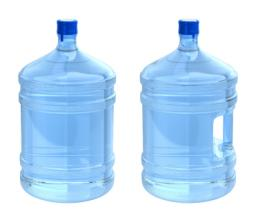We would be pleased if you find an error in the word problem, spelling mistakes, or inaccuracies and send it to us. Thank you!Tips to related online calculators
Do you have a linear equation or system of equations and looking for its solution? Or do you have quadratic equation?
Tip: Our volume units converter will help you with the conversion of volume units.

## Next similar math problems:

• Container with water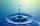The weight of a container with water is 2.48 kg. If the cast 75% water, the container with water has a weight of 0.98 kg. Determine the weight of the empty container. How much water was originally in the container?
• Barrel 3Barrel with water has a weight 118 kg. When we get off 75% of water it has a weight 35 kg. How many kg has empty barrel?
• Barrel 2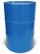Empty barrel weighs 7 kg. When it filled with water up to 45% height, weighs 60 kg. How heavy is barrel full of water?
• Barrel 4Barrel of water weighs 63 kg. After off 75% water, the weight of the barrel with water is 21 kg. How many kg weigh empty barrel and how many kgs water in it?
• Barrel with liquid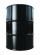Barrel with grain weight 297 kg. When it shed 48% of grain, ha weight 174 kg. What is the weight of empty barrel?
• The water barrel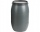The water barrel weighs 122 kg. If we pour 75% of the water out of it, it will weigh 35 kg. What is the weight of the barrel?
• WeightlifterWeightlifter lifted 75% of its weight. Determine how much weight lifted when he weighs 132 kg.
• Barrel of oilBarrel of oil weighs 283 kg. When it mold 26% oil, weighed 216 kg. What is the mass of the empty barrel?
• Barrel with water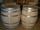The water barrel has a weight of 52 kg. When we pour a quarter of the water, the weight of the barrel is reduced to 40 kg. Determine the weight of the empty barrel.
• Wood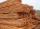Wood contains 12% water. 1 m3 of wood weighs 650 kg. How many liters of water it contains?
• Nectar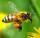Nectar collected by bees contains 70% water. From the nectar of the some process produces honey which contains 19% water. How many kg of nectar bees need to collect to make 1 kg of honey?
• Reservoir + waterReservoir completely filled with water weighs 12 kg. After pouring off three quarters of the amount of water weights 3 kg. Calculate the weight and volume of the reservoir.
• Rain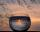How many mm of water rained the roof space 75 m2 if the empty barrel with a radius of 8 dm and height 1.2 m filled to 75% its capacity? :-)
• Cargo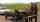Weight of cargo car is 761 kg. Weight of car is 23% of the total weight of car+cargo. What is the weight of the cargo load?
• Drying herbsHerbs lose 75% weight By drying. How many fresh herbs do you have to collect if you want to make 1.5 kg of dried herbs?
• Cargo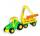Car with cargo weight 7610 kg. Weight of car is 23% of the total weight of car and cargo. How heavy is cargo?
• Three friendsDanica, Lenka and Dalibor have altogether 96 kg. Lenka weighs 75% more than Dalibor and Danica weighs 6 kg more than Dalibor. Determine the weight of Danice, Lenka and Dalibor.